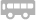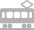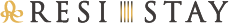组子 Amaterrace

「組子」 “和”的传统与现代之美编织而成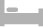房间介绍Hollywood Twin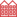公寓式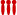可供4位以上入住

[可容纳人数 : 4 位]

• 温水便座
• 浴缸
• 空调
• 冰箱
• 微波炉
• 电水壶
• 電磁爐
• 烹调器具
• 餐具
• TV
• Apple TV
• Netflix
• 吸尘器
• 公用洗衣机
• WiFi
• 保险柜
• 室内禁烟
• 电梯
• 壁橱
• 咖啡 ⁄ 茶 ⁄ 绿茶
• 矿泉水

• 洗发水
• 护发素
• 沐浴液
• 洗脸毛巾
• 浴巾
• 浴室防滑垫
• 拖鞋
• 化妆棉
• 牙刷
• 衣架
• 吹风机
• 化妆棉
• 沐浴剂
• 电熨斗
• 便笺·笔Party Room公寓式可供4位以上入住

[可容纳人数 : 4 位]

• 温水便座
• 浴缸
• 空调
• 冰箱
• 微波炉
• 电水壶
• 電磁爐
• 烹调器具
• 餐具
• TV
• Apple TV
• Netflix
• 吸尘器
• 公用洗衣机
• WiFi
• 保险柜
• 室内禁烟
• 电梯
• 壁橱
• 咖啡 ⁄ 茶 ⁄ 绿茶
• 矿泉水

• 洗发水
• 护发素
• 沐浴液
• 洗脸毛巾
• 浴巾
• 浴室防滑垫
• 拖鞋
• 化妆棉
• 牙刷
• 衣架
• 吹风机
• 化妆棉
• 沐浴剂
• 电熨斗
• 便笺·笔Superior Double Beds公寓式可供4位以上入住

[可容纳人数 : 4 位]

• 温水便座
• 浴缸
• 空调
• 冰箱
• 微波炉
• 电水壶
• 電磁爐
• 烹调器具
• 餐具
• TV
• Apple TV
• Netflix
• 吸尘器
• 公用洗衣机
• WiFi
• 保险柜
• 室内禁烟
• 电梯
• 壁橱
• 咖啡 ⁄ 茶 ⁄ 绿茶
• 矿泉水

• 洗发水
• 护发素
• 沐浴液
• 洗脸毛巾
• 浴巾
• 浴室防滑垫
• 拖鞋
• 化妆棉
• 牙刷
• 衣架
• 吹风机
• 化妆棉
• 沐浴剂
• 电熨斗
• 便笺·笔Standard Family公寓式可供4位以上入住

[可容纳人数 : 5 位]

• 温水便座
• 浴缸
• 空调
• 冰箱
• 微波炉
• 电水壶
• 電磁爐
• 烹调器具
• 餐具
• TV
• Apple TV
• Netflix
• 吸尘器
• 公用洗衣机
• WiFi
• 保险柜
• 室内禁烟
• 电梯
• 壁橱
• 咖啡 ⁄ 茶 ⁄ 绿茶
• 矿泉水

• 洗发水
• 护发素
• 沐浴液
• 洗脸毛巾
• 浴巾
• 浴室防滑垫
• 拖鞋
• 化妆棉
• 牙刷
• 衣架
• 吹风机
• 化妆棉
• 沐浴剂
• 电熨斗
• 便笺·笔Standard fourth公寓式可供4位以上入住

[可容纳人数 : 4 位]

• 温水便座
• 浴缸
• 空调
• 冰箱
• 微波炉
• 电水壶
• 電磁爐
• 烹调器具
• 餐具
• TV
• Apple TV
• Netflix
• 吸尘器
• 公用洗衣机
• WiFi
• 保险柜
• 室内禁烟
• 电梯
• 壁橱
• 咖啡 ⁄ 茶 ⁄ 绿茶
• 矿泉水

• 洗发水
• 护发素
• 沐浴液
• 洗脸毛巾
• 浴巾
• 浴室防滑垫
• 拖鞋
• 化妆棉
• 牙刷
• 衣架
• 吹风机
• 化妆棉
• 沐浴剂
• 电熨斗
• 便笺·笔Superior Family公寓式可供4位以上入住

[可容纳人数 : 5 位]

• 温水便座
• 浴缸
• 空调
• 冰箱
• 微波炉
• 电水壶
• 電磁爐
• 烹调器具
• 餐具
• TV
• Apple TV
• Netflix
• 吸尘器
• 公用洗衣机
• WiFi
• 保险柜
• 室内禁烟
• 电梯
• 壁橱
• 咖啡 ⁄ 茶 ⁄ 绿茶
• 矿泉水

• 洗发水
• 护发素
• 沐浴液
• 洗脸毛巾
• 浴巾
• 浴室防滑垫
• 拖鞋
• 化妆棉
• 牙刷
• 衣架
• 吹风机
• 化妆棉
• 沐浴剂
• 电熨斗
• 便笺·笔[可容纳人数 : 5 位]

• 温水便座
• 浴缸
• 空调
• 冰箱
• 微波炉
• 电水壶
• 電磁爐
• 烹调器具
• 餐具
• TV
• Apple TV
• Netflix
• 吸尘器
• 公用洗衣机
• WiFi
• 保险柜
• 室内禁烟
• 电梯
• 壁橱
• 咖啡 ⁄ 茶 ⁄ 绿茶
• 矿泉水

• 洗发水
• 护发素
• 沐浴液
• 洗脸毛巾
• 浴巾
• 浴室防滑垫
• 拖鞋
• 化妆棉
• 牙刷
• 衣架
• 吹风机
• 化妆棉
• 沐浴剂
• 电熨斗
• 便笺·笔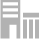设施概要

地址 京都市下京区风早町 566-4 京都市巴士“四条堀川”　B站牌・・・歩行3分钟钟地铁乌丸线“四条”站　25号出口・・・歩行9分钟钟阪急电车“乌丸”站　25号出口・・・歩行9分钟 总计19间 +81-75-353-7744 15:00 10:00 没有 信用卡，Paypal 电梯, WiFi, 洗衣机, 洗衣烘干机交通